# SSAT Elementary Level Math : How to find the area of a square

## Example Questions

1 3 Next →

### Example Question #21 : Geometry

Find the area of the square shown below: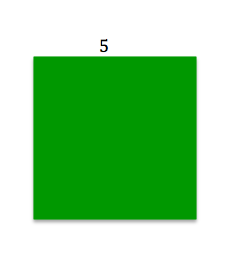Explanation:

A square has sides that are all equal. Area is length times width, and in the case of a square it is the side measurement times itself.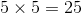### Example Question #22 : Geometry

Use the following to answer the question.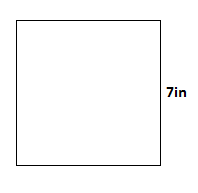Find the area of the square.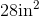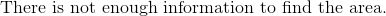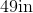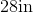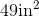Explanation:

To find the area of a square, we use the following formula: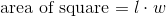where l is the width and w is the width of the square.

If we look at the squarewe can see the width is 7in.  Because it is a square, we know that all sides are equal.  Therefore, the length is also 7in.  Knowing this, we can substitute into the formula.  We get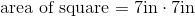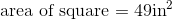### Example Question #23 : Geometry

Use the following square to answer the question: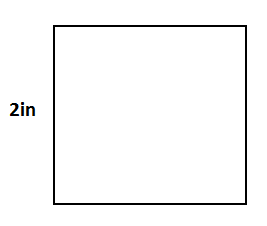Find the area.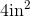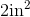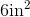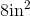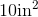Explanation:

To find the area of a square, we will use the following formula:where l is the length and w is the width of the square.

Now, let's look at the square.We can see the width of the square is 2in.  Because it is a square, all sides are equal.  Therefore, the length is also 2in.

Knowing this, we can substitute into the formula.  We get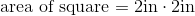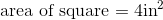### Example Question #24 : Geometry

Use the following square to solve the problem: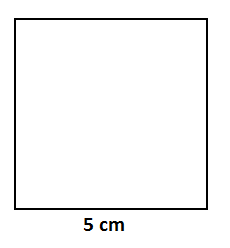Find the area.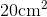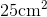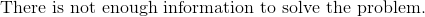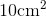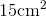Explanation:

To find the area of a square, we will use the following formula: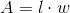where l is the length and w is the width of the square.

Now, given the squarewe can see the length is 5cm.  Because it is a square, all sides are equal.  Therefore, the width is also 5cm.  So, we can substitute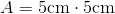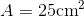1 3 Next →